# Estimating Orientation Using Inertial Sensor Fusion and MPU-9250

This example shows how to get data from an InvenSense MPU-9250 IMU sensor and to use the 6-axis and 9-axis fusion algorithms in the sensor data to compute orientation of the device.

MPU-9250 is a 9-axis sensor with accelerometer,gyroscope, and magnetometer. The accelerometer measures acceleration, the gyroscope measures angular velocity, and the magnetometer measures magnetic field in x-, y- and z- axis. The axis of the sensor depends on the make of the sensor.

### Required MathWorks® Products

• MATLAB®

• MATLAB Support Package for Arduino® Hardware

• Sensor Fusion and Tracking Toolbox™

### Required Hardware

• Arduino Uno

• InvenSense MPU-9250

### Hardware Connection

Connect the SDA, SCL, GND, and the VCC pins of the MPU-9250 sensor to the corresponding pins on the Arduino® Hardware. This example uses the Arduino Uno board with the following connections:

• SDA - A4

• SCL - A5

• VCC - +3.3V

• GND - GND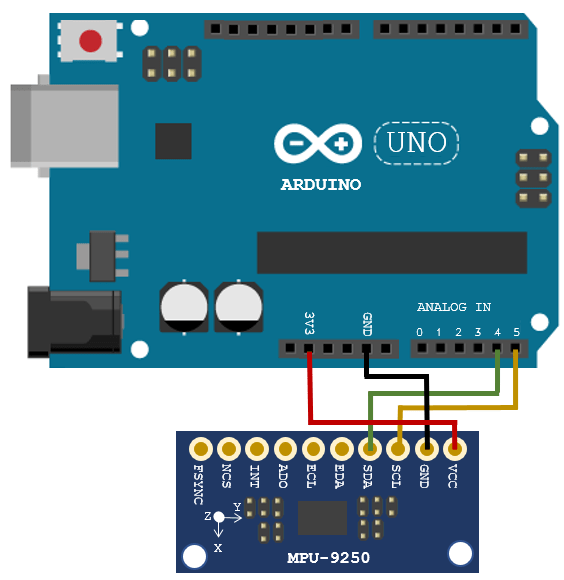Ensure that the connections to the sensors are intact. It is recommended to use a prototype shield and solder the sensor to it to avoid loose connections while moving the sensor. Refer the Troubleshooting Sensors (MATLAB Support Package for Arduino Hardware) page for sensors to debug the sensor related issues.

### Create Sensor Object

Create an arduino object and include the I2C library.

a = arduino('COM9', 'Uno', 'Libraries', 'I2C');
Updating server code on board Uno (COM9). This may take a few minutes. 

Create the MPU-9250 sensor object.

fs = 100; % Sample Rate in Hz imu = mpu9250(a,'SampleRate',fs,'OutputFormat','matrix'); 

### Compensating For Hard Iron Distortions

Fusion algorithms use magnetometer readings which need to compensate for magnetic distortions such as hard iron distortion. Hard iron distortions are produced by materials which create a magnetic field, resulting in shifting the origin on the response surface. These distortions can be corrected by subtracting the correction values from the magnetometer readings for each axis. In order to find the correction values, do the following:

1. Rotate the sensor from 0 to 360 degree around each axis.

2. Obtain the minimum and maximum magnetometer readings.

3. Average the minimum and maximum readings to get the correction values for each axis.

The correction values change with the surroundings.

The following code snippets can be used to obtain bias values for x axis, similar procedure can be followed for other axes as well:

displayMessage(['Fusion algorithms use magnetometer readings which need to compensate for magnetic distortions.' ... 'The given code snippet can be used to find the correction values for compensating Hard Iron Distortions. When the code '... 'is executing, rotate the sensor around x axis from 0 to 360 degree. For other axes, modify the code accordingly and rotate the ' ... 'sensor along that axis'],'Compensating Hard Iron Distortions'); tic; stopTimer = 100; magReadings=[]; while(toc<stopTimer) % Rotate the sensor around x axis from 0 to 360 degree. % Take 2-3 rotations to improve accuracy. % For other axes, rotate around that axis. [accel,gyro,mag] = read(imu); magReadings = [magReadings;mag]; end % For y axis, use magReadings (:,2) and for z axis use magReadings(:,3) magx_min = min(magReadings(:,1)); magx_max = max(magReadings(:,1)); magx_correction = (magx_max+magx_min)/2;

For more accurate tracking, calibrate the magnetometer for other distortions as well. The magcal function (this function is available in the Sensor Fusion and Tracking Toolbox™) can be used to compensate soft iron distortions as well. Change the correction values calculated for your sensor in the readSensorDataMPU9250 function in the example folder.

### Aligning the axis of MPU-9250 sensor with NED Coordinates

Sensor Fusion algorithms used in this example use North-East-Down(NED) as a fixed, parent coordinate system. In the NED reference frame, the X-axis points north, the Y-axis points east, and the Z-axis points down. Depending on the algorithm, north may either be the magnetic north or true north. The algorithms in this example use the magnetic north. The algorithms used here expects all the sensors in the object to have their axis aligned and is in accordance with NED convention.

MPU-9250 has two devices, the magnetometer and the accelerometer-gyroscope, on the same board. The axes of these devices are different from each other. The magnetometer axis is aligned with the NED coordinates. The axis of the accelerometer-gyroscope is different from magnetometer in MPU-9250. The accelerometer and the gyroscope axis need to be swapped and/or inverted to match the magnetometer axis. For more information refer to the section “Orientation of Axes” section in MPU-9250 datasheet.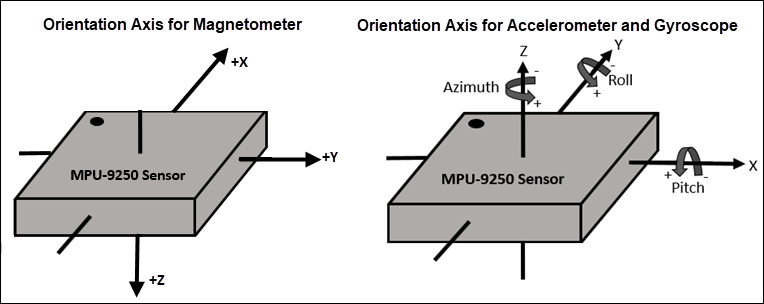To align MPU-9250 accelerometer-gyroscope axes to NED coordinates, do the following:

1. Define device axes: Define the imaginary axis as the device axis on the sensor in accordance to NED coordinate system which may or may not be same as sensor axes. For MPU-9250, magnetometer axis can be considered as device axis.

2. Swap the x and y values of accelerometer and gyroscope readings, so that the accelerometer and gyroscope axis is aligned with magnetometer axis.

3. Determine polarity values for accelerometer, and gyroscope.

a. Accelerometer

• Place the sensor such that device X axis is pointing downwards, perpendicular to the surface at which sensor is kept. Accelerometer readings should read approximately [9.8 0 0]. If not negate the x-values of accelerometer.

• Place the sensor such that device Y axis is pointing downwards, perpendicular to the surface at which sensor is kept. Accelerometer readings should read approximately [0 9.8 0]. If not negate the y-values of accelerometer.

• Place the sensor such that device Z axis is pointing downwards, perpendicular to the surface at which sensor is kept. Accelerometer readings should read approximately [0 0 9.8]. If not negate the z-values of accelerometer.

b. Gyroscope

Rotate the sensor along each axis and capture the readings. Use the right hand screw rule to correct the polarity of rotation.

The above method is used to set the axis of the sensor in this example.

### Tuning Filter Parameters

The algorithms used in this example, when properly tuned, enable estimation of orientation and are robust against environmental noise sources. You must consider the situations in which the sensors are used and tune the filters accordingly. Refer Tuning Filter Parameters for more details related to tuning filter parameters.

The example demonstrates three algorithms to determine orientation, namely ahrsfilter, imufilter, and ecompass. Refer Determine Orientation Using Inertial Sensors for more details related to inertial fusion algorithms.

### Accelerometer-Gyroscope-Magnetometer Fusion

An attitude and heading reference system (AHRS) consist of a 9-axis system that uses an accelerometer, gyroscope, and magnetometer to compute orientation of the device. The ahrsfilter produces a smoothly changing estimate of orientation of the device, while correctly estimating the north direction. The ahrsfilter has the ability to remove gyroscope bias and can also detect and reject mild magnetic jamming.

The following code snippets use ahrsfilter system object to determine orientation of the sensor and creates a figure which gets updated as you move the sensor. The sensor has to be stationary, before the start of this example.

% GyroscopeNoise and AccelerometerNoise is determined from datasheet. GyroscopeNoiseMPU9250 = 3.0462e-06; % GyroscopeNoise (variance value) in units of rad/s AccelerometerNoiseMPU9250 = 0.0061; % AccelerometerNoise(variance value)in units of m/s^2 viewer = HelperOrientationViewer('Title',{'AHRS Filter'}); FUSE = ahrsfilter('SampleRate',imu.SampleRate, 'GyroscopeNoise',GyroscopeNoiseMPU9250,'AccelerometerNoise',AccelerometerNoiseMPU9250); stopTimer = 100;

While the below code is getting executed, slowly move the sensor and check if the motion in the figure matches the motion of the sensor.

% Use ahrsfilter to estimate orientation and update the viewer as the % sensor moves for time specified by stopTimer displayMessage(['This section uses AHRS filter to determine orientation of the sensor by collecting live sensor data from the \slmpu9250 \rm' ... 'system object. Move the sensor to visualize orientation of the sensor in the figure window. Keep the sensor stationery before you' ... 'click OK'],... 'Estimate Orientation using AHRS filter and MPU-9250') tic; while(toc < stopTimer) [accel,gyro,mag] = readSensorDataMPU9250(imu); rotators = FUSE(accel,gyro,mag); for j = numel(rotators) viewer(rotators(j)); end end

When the device X axis of sensor is pointing to north, the device Y-axis is pointing to east and device Z-axis is pointing down.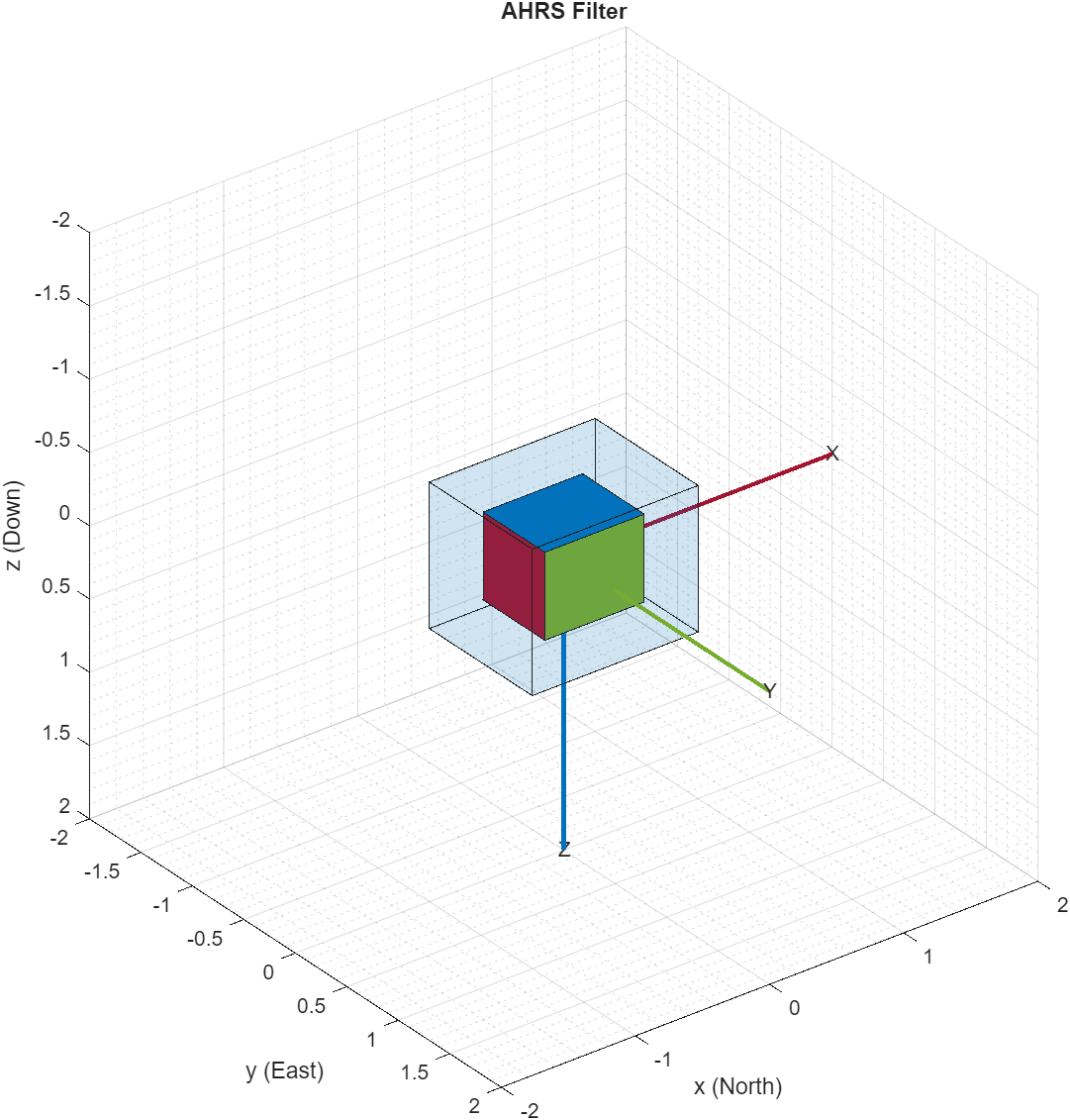When the device X axis of sensor is pointing to north, device Y-axis is pointing to west and device Z-axis is pointing upwards.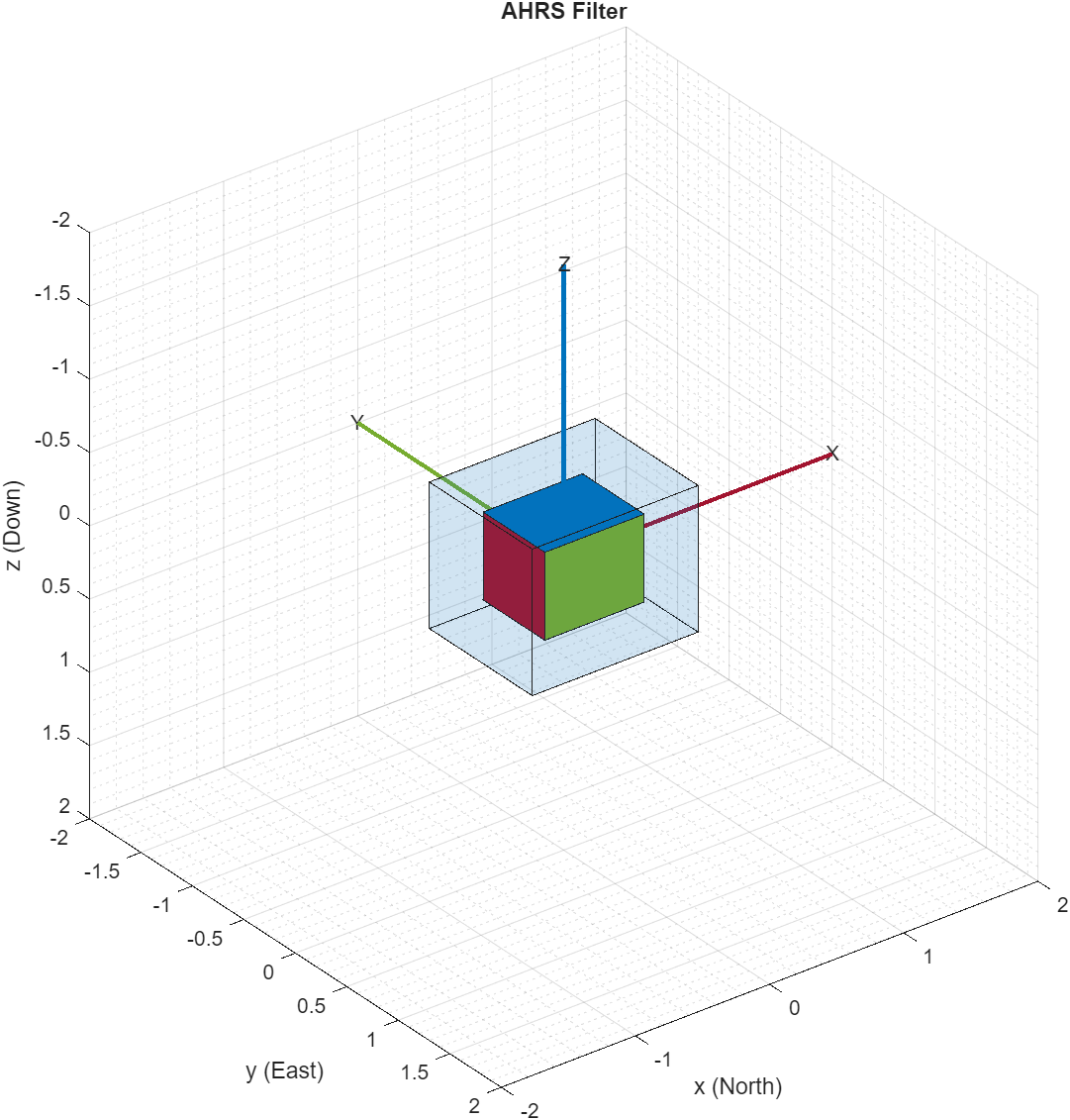### Accelerometer-Gyroscope Fusion

The imufilter system object fuses accelerometer and gyroscope data using an internal error-state Kalman filter. The filter is capable of removing the gyroscope bias noise, which drifts over time. The filter does not process magnetometer data, so it does not correctly estimate the direction of north. The algorithm assumes the initial position of the sensor is in such a way that device X-axis of the sensor is pointing towards magnetic north, the device Y-axis of the sensor is pointing to east and the device Z-axis of the sensor is pointing downwards. The sensor must be stationary, before the start of this example.

The following code snippets use imufilter object to determine orientation of the sensor and creates a figure which gets updated as you move the sensor.

displayMessage(['This section uses IMU filter to determine orientation of the sensor by collecting live sensor data from the \slmpu9250 \rm' ... 'system object. Move the sensor to visualize orientation of the sensor in the figure window. Keep the sensor stationery before you'... 'click OK'],... 'Estimate Orientation using IMU filter and MPU-9250.') % GyroscopeNoise and AccelerometerNoise is determined from datasheet. GyroscopeNoiseMPU9250 = 3.0462e-06; % GyroscopeNoise (variance) in units of rad/s AccelerometerNoiseMPU9250 = 0.0061; % AccelerometerNoise (variance) in units of m/s^2 viewer = HelperOrientationViewer('Title',{'IMU Filter'}); FUSE = imufilter('SampleRate',imu.SampleRate, 'GyroscopeNoise',GyroscopeNoiseMPU9250,'AccelerometerNoise', AccelerometerNoiseMPU9250); stopTimer=100;

While the below code is getting executed, slowly move the sensor and check if the motion in the figure matches the motion of the sensor.

% Use imufilter to estimate orientation and update the viewer as the % sensor moves for time specified by stopTimer tic; while(toc < stopTimer) [accel,gyro] = readSensorDataMPU9250(imu); rotators = FUSE(accel,gyro); for j = numel(rotators) viewer(rotators(j)); end end

The imufilter algorithm can also be used with MPU6050 as well, since it does not require magnetometer values.

When the device X axis of sensor is pointing to north, device Z-axis is pointing downwards and device Y-axis is pointing to east.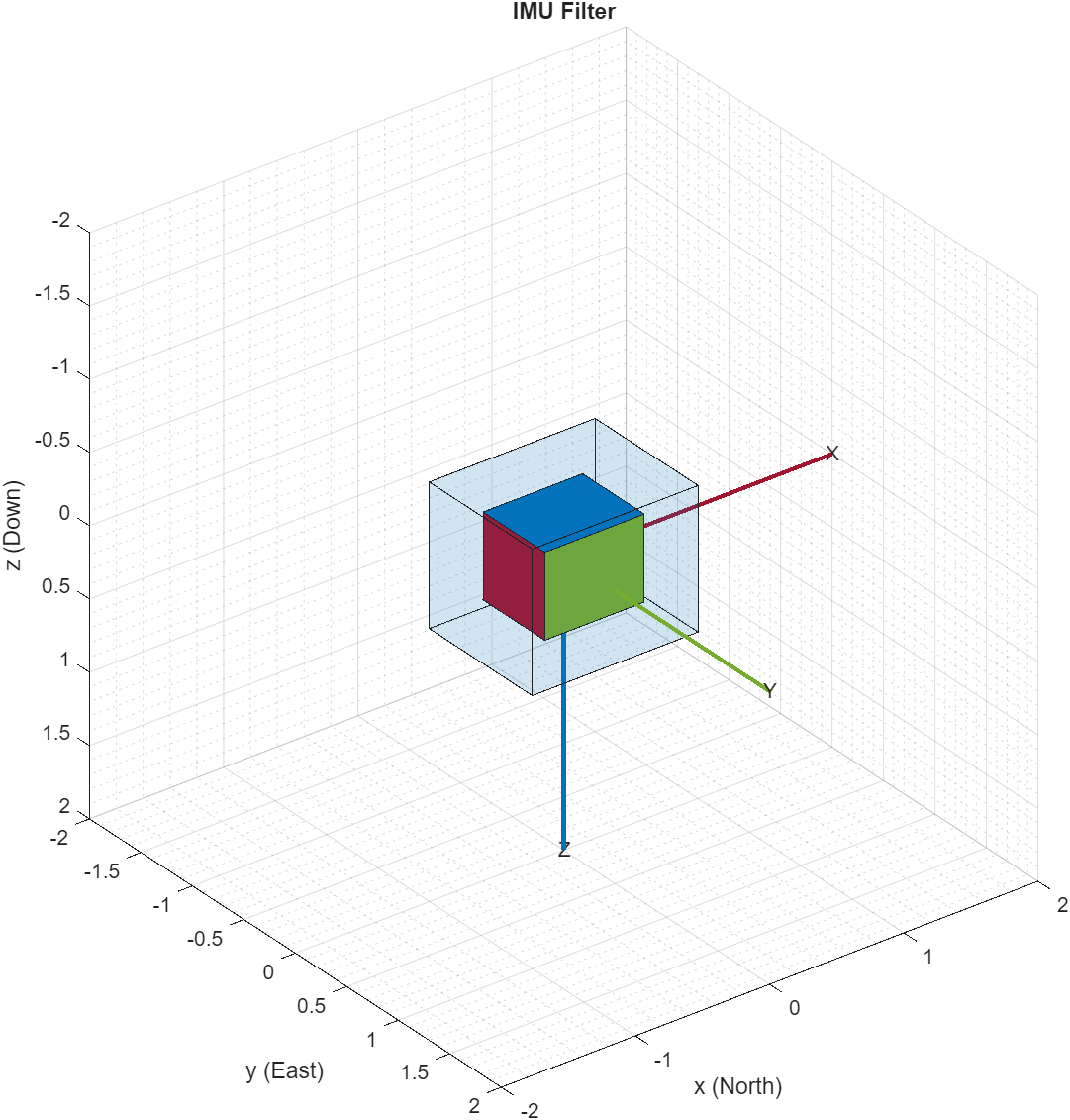When the device X axis of sensor is pointing upwards, device Y-axis is points to west and device Z-axis points to south.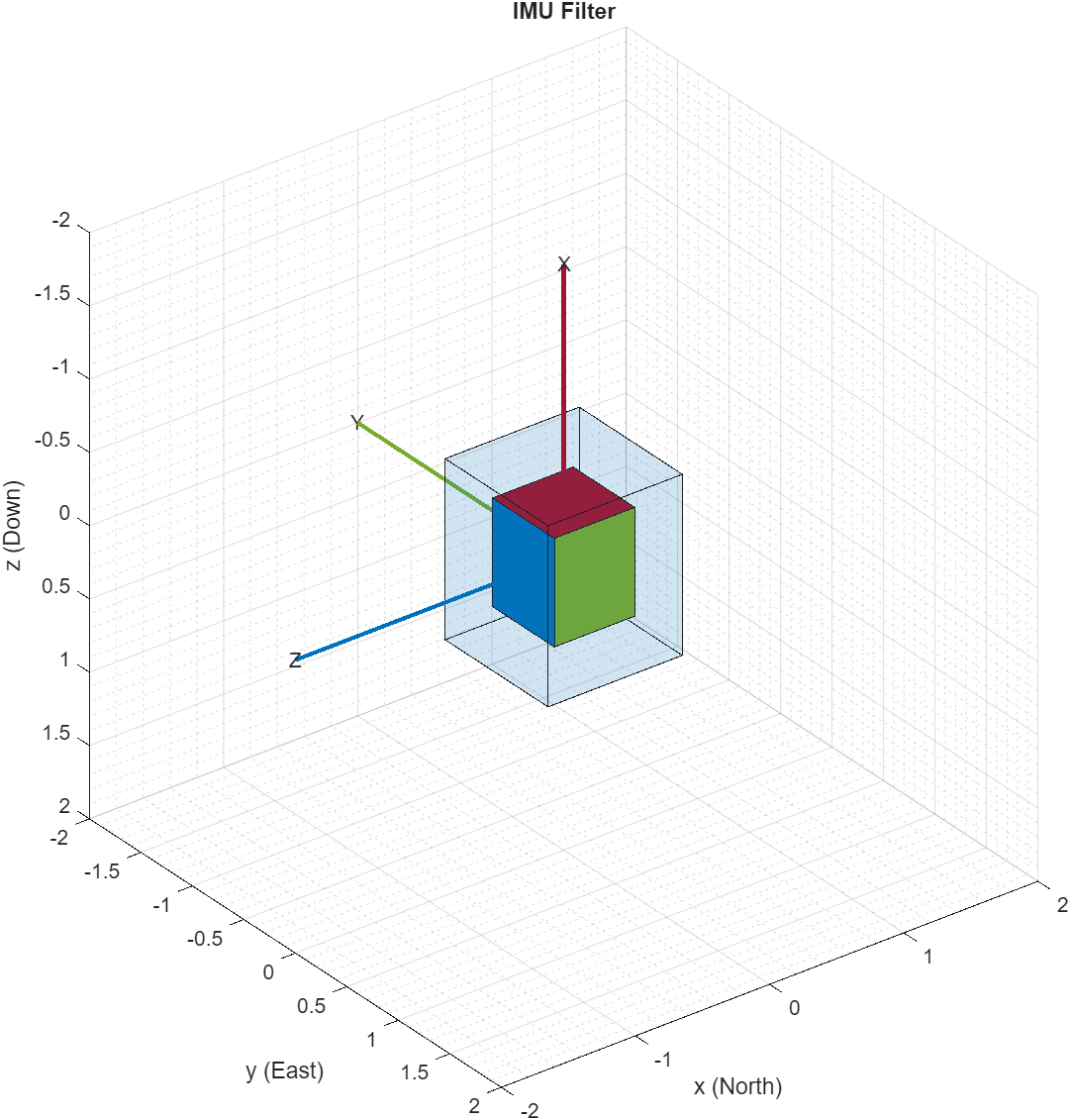### Accelerometer-Magnetometer Fusion

The ecompass system object fuses the accelerometer and magnetometer data. The Ecompass algorithm is a memoryless algorithm that requires no parameter tuning but is highly susceptible to sensor noise. You could use spherical linear interpolation (SLERP) to lowpass filter a noisy trajectory. Refer Lowpass Filter Orientation using Quaternion SLERP example for more details

displayMessage(['This section uses \slecompass \rmfunction to determine orientation of the sensor by collecting live sensor data from the \slmpu9250 ' ... '\rmsystem object. Move the sensor to visualize orientation of the sensor in the figure window. Keep the sensor stationery before you click OK'],... 'Estimate Orientation using Ecompass algorithm.') tic; viewer = HelperOrientationViewer('Title',{'Ecompass Algorithm'}); stopTimer = 100; tic; % Use ecompass algorithm to estimate orientation and update the viewer as the % sensor moves for time specified by stopTimer. while(toc < stopTimer) [accel,gyro,mag] = readSensorDataMPU9250(imu); rotators = ecompass(accel,mag); for j = numel(rotators) viewer(rotators(j)); end end

### Clean Up

When the connection is no longer needed, release and clear the objects

release(imu); delete(imu); clear;

### Things to try

You can try this example with other sensors such as InvenSense MPU-6050 and STMicroelectronics LSM9DS1. Note that the MPU-6050 sensor can be used only with the imufilter system object.

##### SupportGet trial now